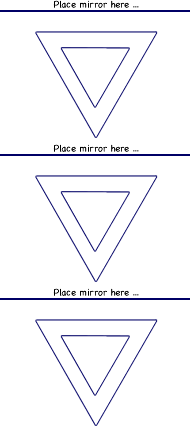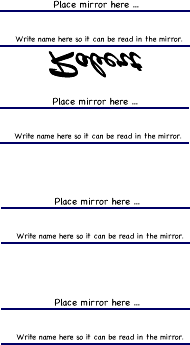Reaction Time, Practice, and Learning

Some interesting facts.

1. We process sound faster than sight.
2. A touch to the foot takes longer to react to than a touch to the hand.
3. What we experience as reality and real time is 5 milliseconds behind what happens and our experience of it.

Counting to 1016 in base sixteen1. See how fast you can complete the task and record the times.
2. Record the first three and the last if you do it more than four times.
3. Try it again tomorrow after you sleep on it and record those times.
Time data by trials and comparison Time in seconds
Day 1 - trial 1
Day 1 - trial 2
Day 1 - trial 3
Day 1 - trial last
Difference between Day 1 trial 1
and Day 1 trial last

Day 2 - trial 1
Day 2 - trial 2
Day 2 - trial 3
Day 2 - trial last
Difference between Day 2 trial 1
and Day 2 trial last

Difference between Day 1 best time
and Day 2 best time

Follow the path in the mirror

Materials: mirror, notebook or folder, and work sheet.

Procedure:

1. Stand a mirror on the writing that says: Place mirror here. So you can see the triangles in the mirror.
2. Have someone hold a notebook or folder between your eyes and the paper so the triangles can only be seen in the mirror.
3. Hold your hands in the air until the person keeping the time says go. Draw a closed path by looking in the mirror. A closed path that goes all the way around the inner triangle so it is between the lines of the two triangles and returns to the start with out touching or crossing the lines of either triangle.Time data by trials and comparison Time in seconds
Day 1 - trial 1
Day 1 - trial 2
Day 1 - trial 3
Difference between slowest and best times
Day 2 - trial 1
Day 2 - trial 2
Day 2 - trial 3
Difference between slowest and best times
Difference between Day 1 best and Day 2 best

Mirror Writing

Materials: mirror and work sheet.

Procedure: place a mirror on the line that says, "Mirror here:" Have someone time how long it takes to write your name on the line so it can be read in the mirror. See sample below.Time data by trials and comparison Time in seconds
Day 1 - trial 1
Day 1 - trial 2
Day 1 - trial 3
Difference between Day 1 trial 1
and Day 1 trial last

Day 2 - trial 1
Day 2 - trial 2
Day 2 - trial 3
Day 2 - trial last
Difference between Day 2 trial 1
and Day 2 trial last

Difference between Day 1 best time
and Day 2 best time

Reaction time and signal response time through the body

Here is a problem to investigate and discover information about reaction time and the time it takes a nerve signal to move through the body.

If reaction time is how long it takes to respond to a stimulus, then what is the reaction time and what is the time it takes for the signal to travel along a path to the brain and back to a body part.

To find out gather a chain of 10 or 20 people and have them line up so each person can put one hand placed on the back of the shoulder of the person in front of them.

Then have a person time how long it will take to pass a signal up the line by having the timer say go so the person at back of the line can begin by slightly pressing on the person's shoulder in front of them. When that person feels it, they press on the person in front of them and so on until the person at the other end of the line feels their shoulder being touched and says stop. The timer can then tell how long it took the signal to move through the line.

The time can be divided by the number of people in the line to find an average for how long it takes the signal to move through the body and how long it takes to respond.

To see how the time might increase with a longer route the experiment can be repeated with the same line of people, but this time increase the distance the signal has to travel by having the people in the line bend down and touch the back ankle of the person in front of them.

The time it takes this time will include the reaction time and the increased distance from the ankle to the back.

With a little math the time it takes the signal to travel one meter through the body can be determined.

Of course the procedure could be repeated several times to get an average to use to increase the accuracy.

Enjoy!

Reaction Time Ruler Test

Materials: meter stick, notebook or folder, calculator, and gravity.

Procedure:

1. The person, whose reaction time is being test, sits at the edge of a table, resting their elbow on the table so their wrist extends over the side of the table with their hand positioned to catch the meter stick as soon as it is dropped.
2. Another person holds a meter stick vertically above the subject's thumb and index finger so the zero edge of the meter stick is close, but not touching.
3. When ready, drop the meter stick without warning.
4. Record the centimeters the ruler fell before it was caught.
5. Repeat several times (e.g. 10 times) and find the average distance the meter stick dropped before it was caught.
6. Use the table below to determine the time in seconds it took the ruler to fall over the measured distance in centimeters (cm).
7. The formula: t = sqrt ( 2d / g ) is used to calculate time. d = the distance the ruler fell in meters, g = the acceleration of gravity in meters (9.8 m/s2), and t = the time the ruler was falling in seconds.

1 cm = 0.045 s, 26 cm = 0.230 s, 51 cm = 0.323 s, 76 cm = 0.394 s,
2 cm = 0.064 s, 27 cm = 0.235 s, 52 cm = 0.326 s, 77 cm = 0.396 s,
3 cm = 0.078 s, 28 cm = 0.239 s, 53 cm = 0.329 s, 78 cm = 0.399 s,
4 cm = 0.090 s, 29 cm = 0.243 s, 54 cm = 0.332 s, 79 cm = 0.402 s,
5 cm = 0.101 s, 30 cm = 0.247 s, 55 cm = 0.335 s, 80 cm = 0.404 s,
6 cm = 0.111 s, 31 cm = 0.252 s, 56 cm = 0.338 s, 81 cm = 0.407 s,
7 cm = 0.120 s, 32 cm = 0.256 s, 57 cm = 0.341 s, 82 cm = 0.409 s,
8 cm = 0.128 s, 33 cm = 0.260 s, 58 cm = 0.344 s, 83 cm = 0.412 s,
9 cm = 0.136 s, 34 cm = 0.263 s, 59 cm = 0.347 s, 84 cm = 0.414 s,
10 cm = 0.143 s, 35 cm = 0.267 s, 60 cm = 0.350 s, 85 cm = 0.416 s,
11 cm = 0.150 s, 36 cm = 0.271 s, 61 cm = 0.353 s, 86 cm = 0.419 s,
12 cm = 0.156 s, 37 cm = 0.275 s, 62 cm = 0.356 s, 87 cm = 0.421 s,
13 cm = 0.163 s, 38 cm = 0.278 s, 63 cm = 0.359 s, 88 cm = 0.424 s,
14 cm = 0.169 s, 39 cm = 0.282 s, 64 cm = 0.361 s, 89 cm = 0.426 s,
15 cm = 0.175 s, 40 cm = 0.286 s, 65 cm = 0.364 s, 90 cm = 0.429 s,
16 cm = 0.181 s, 41 cm = 0.289 s, 66 cm = 0.367 s, 91 cm = 0.431 s,
17 cm = 0.186 s, 42 cm = 0.293 s, 67 cm = 0.370 s, 92 cm = 0.433 s,
18 cm = 0.192 s, 43 cm = 0.296 s, 68 cm = 0.373 s, 93 cm = 0.436 s,
19 cm = 0.197 s, 44 cm = 0.300 s, 69 cm = 0.375 s, 94 cm = 0.438 s,
20 cm = 0.202 s, 45 cm = 0.303 s, 70 cm = 0.378 s, 95 cm = 0.440 s,
21 cm = 0.207 s, 46 cm = 0.306 s, 71 cm = 0.381 s, 96 cm = 0.443 s,
22 cm = 0.212 s, 47 cm = 0.310 s, 72 cm = 0.383 s, 97 cm = 0.445 s,
23 cm = 0.217 s, 48 cm = 0.313 s, 73 cm = 0.386 s, 98 cm = 0.447 s,
24 cm = 0.221 s, 49 cm = 0.316 s, 74 cm = 0.389 s, 99 cm = 0.449 s,
25 cm = 0.226 s, 50 cm = 0.319 s, 75 cm = 0.391 s, 100 cm = 0.452 s

This test can be used to determine the reaction time for different situations. For example to a sound. The Apparatus can be used with the subject wearing a blindfold, and the meter stick being released at the same time as an auditory signal.